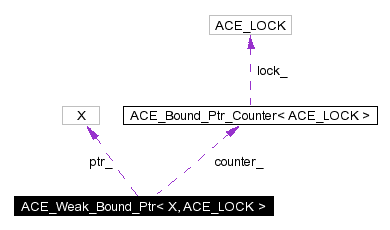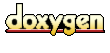Main Page   Namespace List   Class Hierarchy   Alphabetical List   Compound List   File List   Namespace Members   Compound Members   File Members   Related Pages

# ACE_Weak_Bound_Ptr< X, ACE_LOCK > Class Template Reference

This class implements support for a weak pointer that complements ACE_Strong_Bound_Ptr. More...

`#include <Bound_Ptr.h>`

Collaboration diagram for ACE_Weak_Bound_Ptr< X, ACE_LOCK >:[legend]
List of all members.

## Public Methods

ACE_EXPLICIT ACE_Weak_Bound_Ptr (X *p=0)
ACE_Weak_Bound_Ptr (const ACE_Weak_Bound_Ptr< X, ACE_LOCK > &r)
Copy constructor binds <this> and <r> to the same object.

ACE_Weak_Bound_Ptr (const ACE_Strong_Bound_Ptr< X, ACE_LOCK > &r)
Constructor binds <this> and <r> to the same object.

~ACE_Weak_Bound_Ptr (void)
Destructor.

void operator= (const ACE_Weak_Bound_Ptr< X, ACE_LOCK > &r)
Assignment operator that binds <this> and <r> to the same object.

void operator= (const ACE_Strong_Bound_Ptr< X, ACE_LOCK > &r)
Assignment operator that binds <this> and <r> to the same object.

int operator== (const ACE_Weak_Bound_Ptr< X, ACE_LOCK > &r) const
int operator== (const ACE_Strong_Bound_Ptr< X, ACE_LOCK > &r) const
int operator== (X *p) const
int operator!= (const ACE_Weak_Bound_Ptr< X, ACE_LOCK > &r) const
Inequality operator, which is the opposite of equality.

int operator!= (const ACE_Strong_Bound_Ptr< X, ACE_LOCK > &r) const
Inequality operator, which is the opposite of equality.

int operator!= (X *p) const
Inequality operator, which is the opposite of equality.

ACE_Strong_Bound_Ptr< X, ACE_LOCK > operator-> (void) const
Redirection operator.

ACE_Strong_Bound_Ptr< X, ACE_LOCK > strong (void) const
X * unsafe_get (void) const
void reset (X *p=0)
Increment the reference count on the underlying object.

int remove_ref (void)
int null (void) const
Allows us to check for NULL on all ACE_Weak_Bound_Ptr objects.

## Public Attributes

ACE_ALLOC_HOOK_DECLARE
Declare the dynamic allocation hooks.

## Private Types

typedef ACE_Bound_Ptr_Counter<
ACE_LOCK >
COUNTER
The ACE_Bound_Ptr_Counter type.

## Private Attributes

COUNTERcounter_
The reference counter.

X * ptr_
The underlying object.

## Friends

class ACE_Strong_Bound_Ptr< X, ACE_LOCK >

## Detailed Description

### template<class X, class ACE_LOCK> class ACE_Weak_Bound_Ptr< X, ACE_LOCK >

This class implements support for a weak pointer that complements ACE_Strong_Bound_Ptr.

Unlike ACE_Strong_Bound_Ptr, assigning or copying instances of an ACE_Weak_Bound_Ptr will not automatically increment the reference count of the underlying object. What ACE_Weak_Bound_Ptr does is preserve the knowledge that the object is in fact reference counted, and thus provides an alternative to raw pointers where non-ownership associations must be maintained. When the last instance of an ACE_Strong_Bound_Ptr that references a particular object is destroyed or overwritten, the corresponding ACE_Weak_Bound_Ptr instances are set to NULL.

## Member Typedef Documentation

 template typedef ACE_Bound_Ptr_Counter ACE_Weak_Bound_Ptr< X, ACE_LOCK >::COUNTER` [private]`
 The ACE_Bound_Ptr_Counter type.

## Constructor & Destructor Documentation

 template ACE_Weak_Bound_Ptr< X, ACE_LOCK >::ACE_Weak_Bound_Ptr ( X * p = 0 ) ` [inline]`
 Constructor that initializes an ACE_Weak_Bound_Ptr to point to the object

immediately.

 template ACE_Weak_Bound_Ptr< X, ACE_LOCK >::ACE_Weak_Bound_Ptr ( const ACE_Weak_Bound_Ptr< X, ACE_LOCK > & r ) ` [inline]`
 Copy constructor binds and to the same object.

 template ACE_Weak_Bound_Ptr< X, ACE_LOCK >::ACE_Weak_Bound_Ptr ( const ACE_Strong_Bound_Ptr< X, ACE_LOCK > & r ) ` [inline]`
 Constructor binds and to the same object.

 template ACE_Weak_Bound_Ptr< X, ACE_LOCK >::~ACE_Weak_Bound_Ptr ( void ) ` [inline]`
 Destructor.

## Member Function Documentation

 template int ACE_Weak_Bound_Ptr< X, ACE_LOCK >::add_ref ( void ) ` [inline]`
 Increment the reference count on the underlying object. Returns the new reference count on the object. This function may be used to integrate the bound pointers into an external reference counting mechanism such as those used by COM or CORBA servants.

 template int ACE_Weak_Bound_Ptr< X, ACE_LOCK >::null ( void ) const` [inline]`
 Allows us to check for NULL on all ACE_Weak_Bound_Ptr objects.

 template int ACE_Weak_Bound_Ptr< X, ACE_LOCK >::operator!= ( X * p ) const` [inline]`
 Inequality operator, which is the opposite of equality.

 template int ACE_Weak_Bound_Ptr< X, ACE_LOCK >::operator!= ( const ACE_Strong_Bound_Ptr< X, ACE_LOCK > & r ) const` [inline]`
 Inequality operator, which is the opposite of equality.

 template int ACE_Weak_Bound_Ptr< X, ACE_LOCK >::operator!= ( const ACE_Weak_Bound_Ptr< X, ACE_LOCK > & r ) const` [inline]`
 Inequality operator, which is the opposite of equality.

 template ACE_Strong_Bound_Ptr< X, ACE_LOCK > ACE_Weak_Bound_Ptr< X, ACE_LOCK >::operator-> ( void ) const` [inline]`
 Redirection operator. It returns a temporary strong pointer and makes use of the chaining properties of operator-> to ensure that the underlying object does not disappear while you are using it. If you are certain of the lifetimes of the object, and do not want to incur the locking overhead, then use the unsafe_get method instead.

 template void ACE_Weak_Bound_Ptr< X, ACE_LOCK >::operator= ( const ACE_Strong_Bound_Ptr< X, ACE_LOCK > & r ) ` [inline]`
 Assignment operator that binds and to the same object.

 template void ACE_Weak_Bound_Ptr< X, ACE_LOCK >::operator= ( const ACE_Weak_Bound_Ptr< X, ACE_LOCK > & r ) ` [inline]`
 Assignment operator that binds and to the same object.

 template int ACE_Weak_Bound_Ptr< X, ACE_LOCK >::operator== ( X * p ) const` [inline]`
 Equality operator that returns 1 if the ACE_Weak_Bound_Ptr and the raw pointer point to the same underlying object.

 template int ACE_Weak_Bound_Ptr< X, ACE_LOCK >::operator== ( const ACE_Strong_Bound_Ptr< X, ACE_LOCK > & r ) const` [inline]`
 Note: It also returns 1 if both objects have just been instantiated and not used yet.

 template int ACE_Weak_Bound_Ptr< X, ACE_LOCK >::operator== ( const ACE_Weak_Bound_Ptr< X, ACE_LOCK > & r ) const` [inline]`
 Note: It also returns 1 if both objects have just been instantiated and not used yet.

 template int ACE_Weak_Bound_Ptr< X, ACE_LOCK >::remove_ref ( void ) ` [inline]`
 Returns the new reference count on the object. This function may be used to integrate the bound pointers into an external reference counting mechanism such as those used by COM or CORBA servants.

 template void ACE_Weak_Bound_Ptr< X, ACE_LOCK >::reset ( X * p = 0 ) ` [inline]`
 Resets the ACE_Weak_Bound_Ptr to refer to a different underlying object.

 template ACE_Strong_Bound_Ptr< X, ACE_LOCK > ACE_Weak_Bound_Ptr< X, ACE_LOCK >::strong ( void ) const` [inline]`
 Obtain a strong pointer corresponding to this weak pointer. This function is useful to create a temporary strong pointer for conversion to a reference.

 template X * ACE_Weak_Bound_Ptr< X, ACE_LOCK >::unsafe_get ( void ) const` [inline]`
 Get the pointer value. Warning: this does not affect the reference count of the underlying object, so it may disappear on you while you are using it if you are not careful.

## Friends And Related Function Documentation

 template friend class ACE_Strong_Bound_Ptr< X, ACE_LOCK >` [friend]`

## Member Data Documentation

 template ACE_Weak_Bound_Ptr< X, ACE_LOCK >::ACE_ALLOC_HOOK_DECLARE
 Declare the dynamic allocation hooks.

 template COUNTER* ACE_Weak_Bound_Ptr< X, ACE_LOCK >::counter_` [private]`
 The reference counter.

 template X* ACE_Weak_Bound_Ptr< X, ACE_LOCK >::ptr_` [private]`
 The underlying object.

The documentation for this class was generated from the following files:
Generated on Fri Apr 2 16:55:30 2004 for ACE by1.2.18